# Problem 58941. Compute measurement uncertainty

Suppose a variable y depends on n independent variables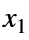,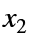,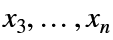. If the independent variables have uncertainty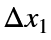,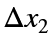, etc. and the uncertainties are independent, then the uncertainty in y can be estimated withFor this problem, the relationship between y and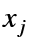will be power laws of the form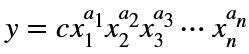For example, the relationshipwould have c = 0.5 and a = [1 2].
Write a function that takes a vector of values of x, the coefficient c, exponents a, and a vector of uncertainties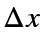and returns the absolute uncertainty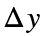, relative uncertainty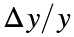, and the index of the variable that contributes the most to the uncertainty in y. Identifying the largest contributor to the uncertainty tells the experimentalist which measurement to target first to improve the measurement.

### Solution Stats

19.44% Correct | 80.56% Incorrect
Last Solution submitted on Sep 26, 2023

### Community Treasure Hunt

Find the treasures in MATLAB Central and discover how the community can help you!

Start Hunting!